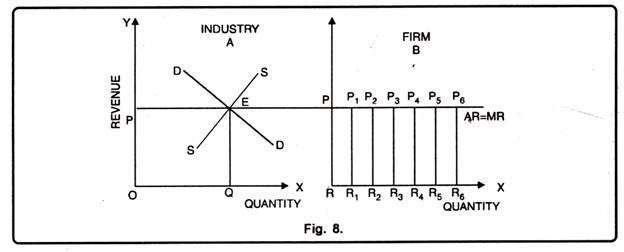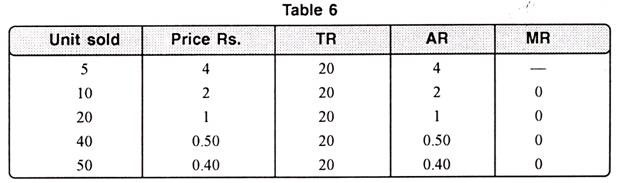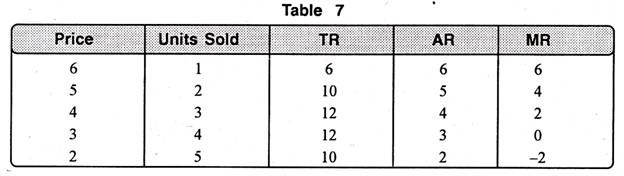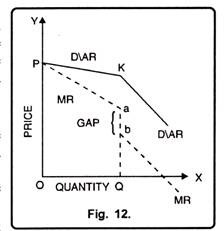# Revenue Curves

### (i) Revenue Curve under Perfect competition

Perfect competition is the term applied to a situation in which the individual buyer or seller (firm) represent such a small share of the total business transacted in the market that he exerts no perceptible influence on the price of the commodity in which he deals.

Thus, in perfect competition an individual firm is price taker, because the price is determined by the collective forces of market demand and supply which are not influenced by the individual. When price is the same for all units of a commodity, naturally AR (Price) will be equal to MR i.e., AR = MR. The revenue schedule for a competitive firm is shown in the table 5.In table 5 we find that as output increases, AR remains the same i.e. Rs. 5. Total revenue increases but at a constant rate. Marginal revenue is also constant i.e. Rs. 5 and is equal to AR.

Thus

TR = AR x Q

Also TR = MR x Q [Since AR = MR]

In figure 8, on the X-axis, we take quantity whereas on Y-axis, we take revenue. At price OP, the seller can sell any amount of the commodity. In this case the average revenue curve is the horizontal line. The Marginal Revenue curve coincides with the Average Revenue.

It is because additional units are sold at the same price as before. In that case AR = MR. A noteworthy point is that OP price is determined by demand and supply of industry.

The firm only follows, (see figure below)### (ii) Revenue Curves under Monopoly

Monopoly is opposite to perfect competition. Under monopoly both AR and MR curves slope downward. It indicates that to sell more units of a commodity, the monopolist will have to lower the price. This can be shown with the help of table 6.In case of pure monopoly, AR curve can be rectangular hyperbola as has been shown in Fig. 9. In this situation, a producer is so powerful that by selling his output at different prices, he can make the consumer spend his income on the concerned commodity. In this case AR curve is rectangular hyperbola. It implies that TR of the monopolist will remain same whatever may be the price. Area below each point of AR curve will be equal to each other. When TR is constant MR curve will be represented by OX-axis as has been shown in figure 9.### (iii) Revenue Curve under Imperfect Competition

When a firm is working under conditions of monopoly or imperfect competition, its demand curve or AR curve is less than perfectly elastic, the exact degree of elasticity being different in different market situations depending upon the number of sellers and the nature of product.

In other words, the demand/AR curve has a negative slope and the MR curve lies below it. This is because the monopolist seller ordinarily has to accept a lower price for his product, as he increases his sales.

Under imperfect competition conditions, total revenue increases at a diminishing rate. It becomes maximum and then begins to decline.

The position of various revenue curves is shown in Table 7In table 7, 2 units can be sold at a unit price of Rs. 5, bringing in total revenue of Rs. 10. When 3 units are sold, the price per unit is lowered to Rs. 4 to make it possible for larger quantity to be sold. The total revenue in this case is Rs. 12.

The marginal unit is not bringing in Rs. 4 which is its price, but only Rs. 2. This is because the additional one unit is sold at Re. one less and the first 2 units which could have been sold for Rs. 5 are also sold at Rs. 4. i.e., Re. one less.

Fig. 10 A shows that as additional units are sold when price comes down not only for the marginal units but also for other previous units. As a result, marginal units do not bring revenue equal to its price. In fig. 10 B. TR increases at a diminishing rate, becomes maximum at point N and then begins to decline. This has been represented by the curve TR. AR at any point on the TR curve is given by the slope of straight line joining the point to the origin. For instance, AR at any point N on TR curve is given by the slope of line### (iv) Revenue Curves under Oligopoly

Under oligopoly market situation the number of sellers is small. The price reduction or extension by one firm affects the other firms. If a seller raises the price of his product, others will not follow him. They know that by following the same price, they can earn more profits. That producer, who has raised the price, is likely to suffer losses because demand of his product will fall.In this case, as shown in Fig. 11, the AR curve becomes highly elastic after K whereas it was less elastic before K. MR, corresponding to AR curve rises discontinuously from b. After that it again takes its course at a new higher level.

(b) If a firm has a kinked demand curve i.e. when it expects that other firms will follow, then it will cut the price. In that case MR curve will be discontinuous at the point of the kink. This can be shown with the help of a Fig. 12.

If under oligopoly, a seller reduces the price of his product; his rivals also follow him in reducing the price of their product. If it is done so, he may not be in a position to raise his sales. Thus AR curve becomes less elastic from K onwards and correspondingly MR curve falls vertically from a to b and then slopes at a lower level.

Thus, from the above analysis we can conclude that:

1. Under perfect competition, average revenue curve is a straight horizontal line and is equal to MR.

2. In pure monopoly, AR curve is a rectangular hyperbola and MR curve coincides with the horizontal axis.

3. In all other markets, AR curve slopes downwards and MR curve lies below it. In oligopoly, however, AR curve cannot be drawn with definiteness but the practice is to draw downward sloping AR and MR curves.

## 3 thoughts on “Revenue Curves”

error: Content is protected !!